•Gurobi Staff

Hi Fernanda,

Please note that it is not possible to reproduce the error from the code snippet you provided, because the call of the $$\texttt{max_flow}$$ function is missing.

The error you see

  (gp.quicksum( flow[h, y, x] for x in leavingnode) - gp.quicksum(KeyError: (u'instagram', 1.0, 1.0)

states that there is no object $$\texttt{flow[u'instagram', 1.0, 1.0]}$$.

Best regards,
Jaromił

•Hi Jaromil,

thank you for reaching out! I do make a call to the function. Let me add the whole code and screenshots of the spreadsheet. I believe the problem is the flow conservation part because if I remove that part of the code then at least the capacities print out.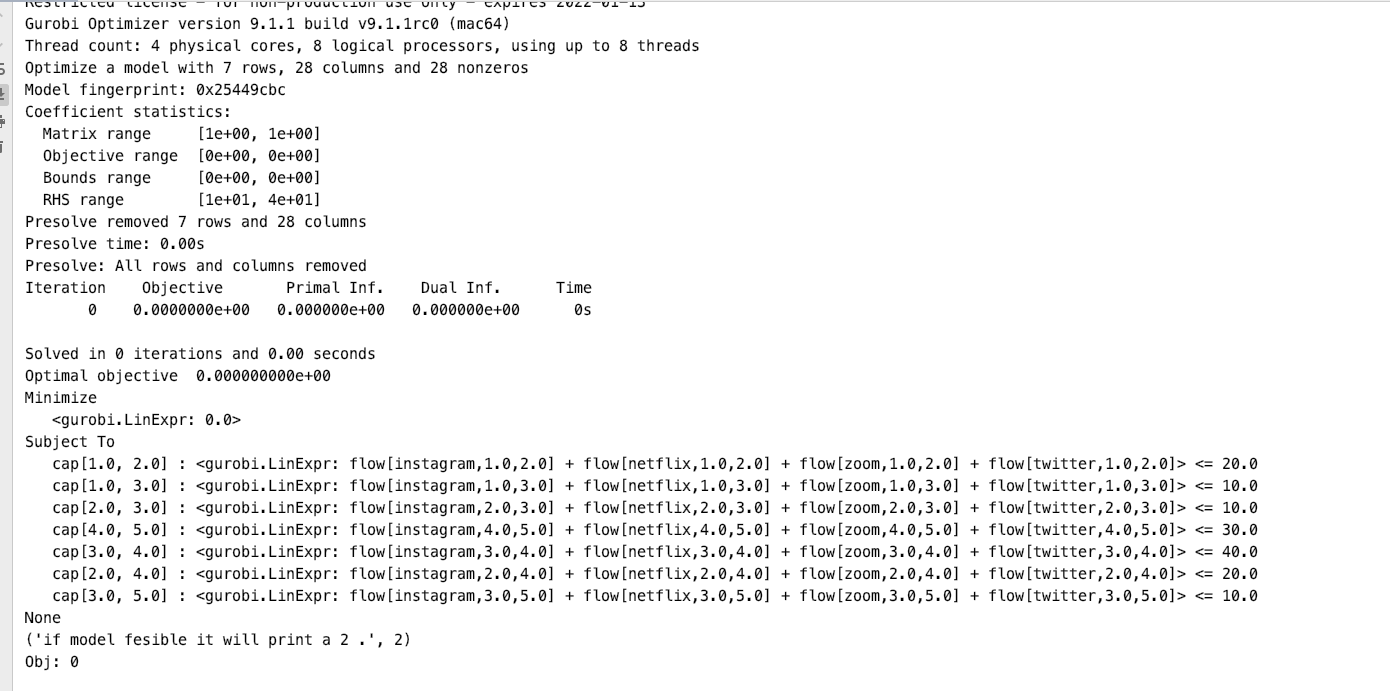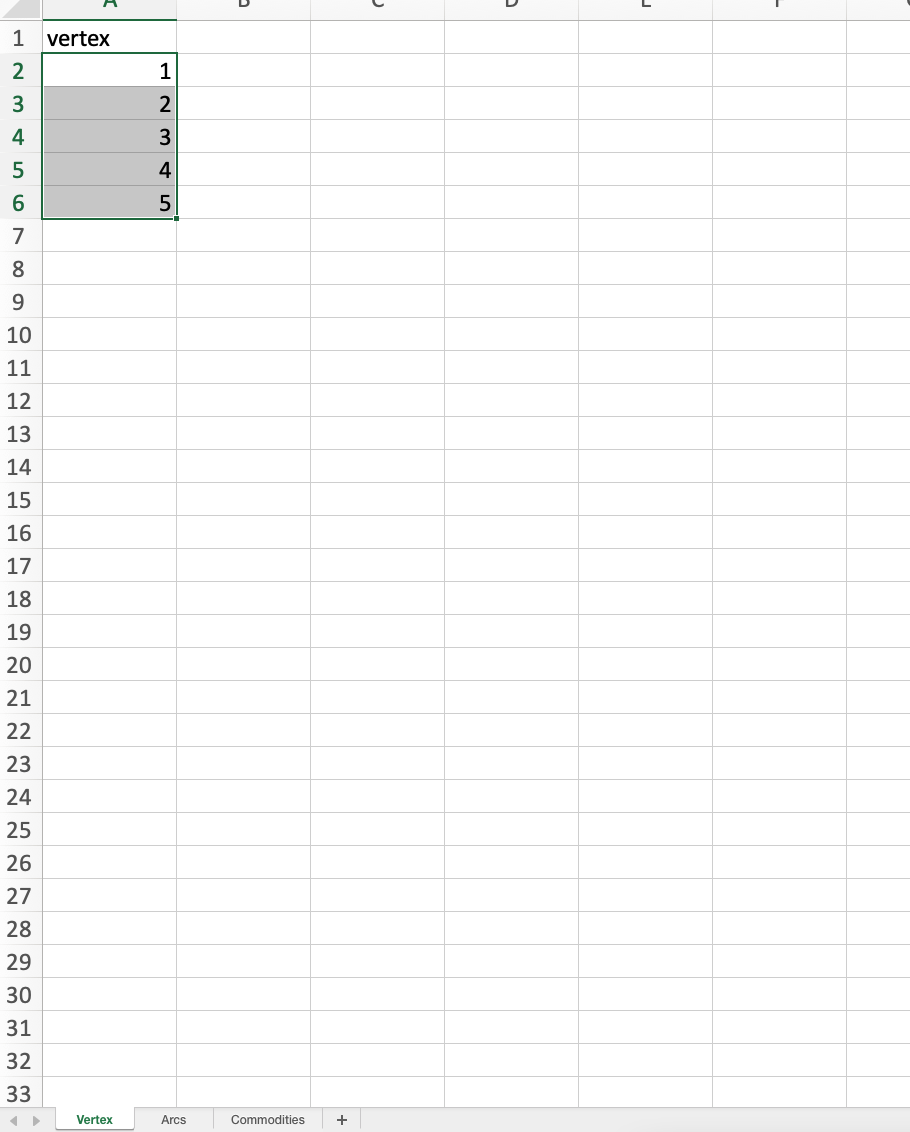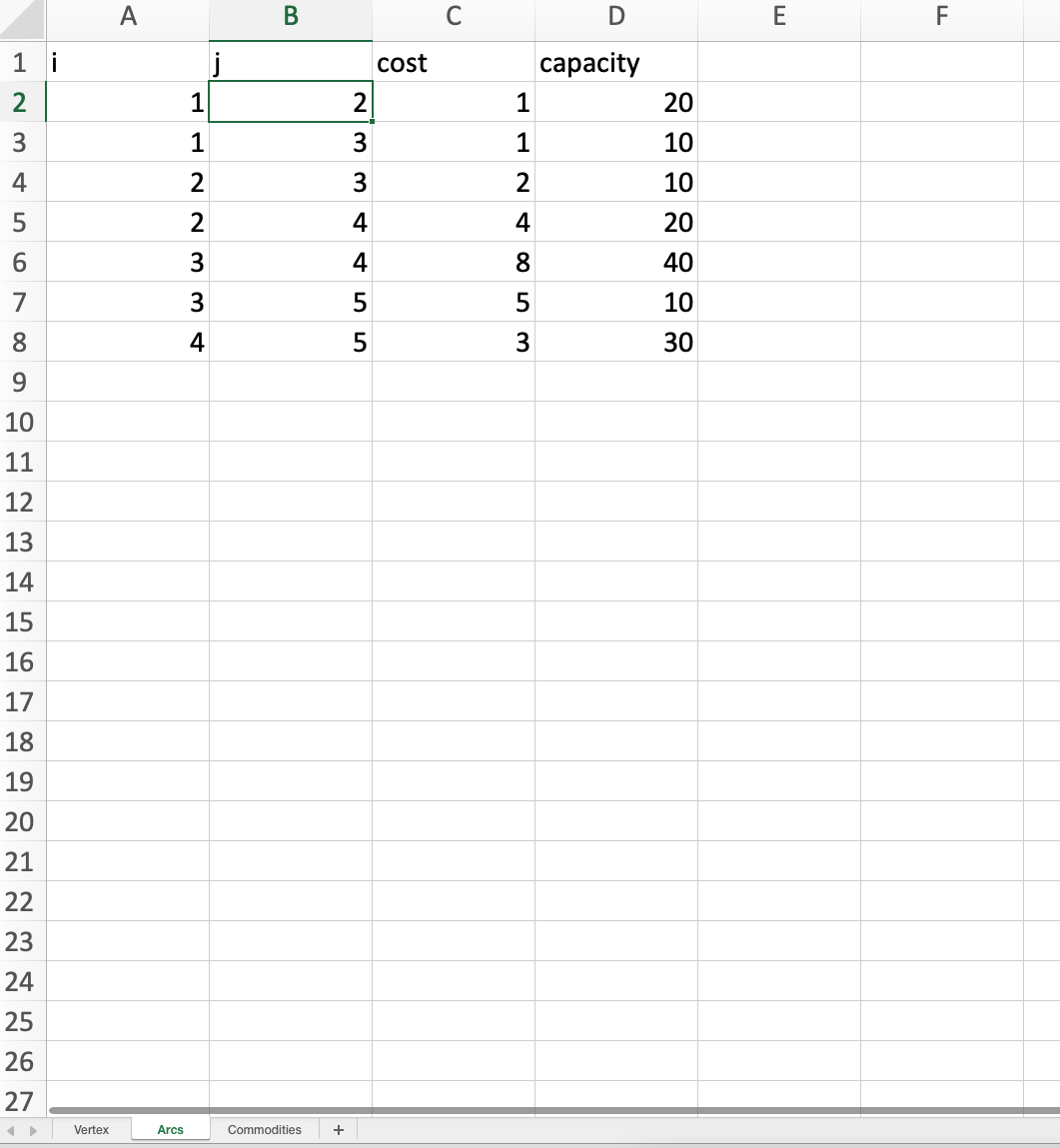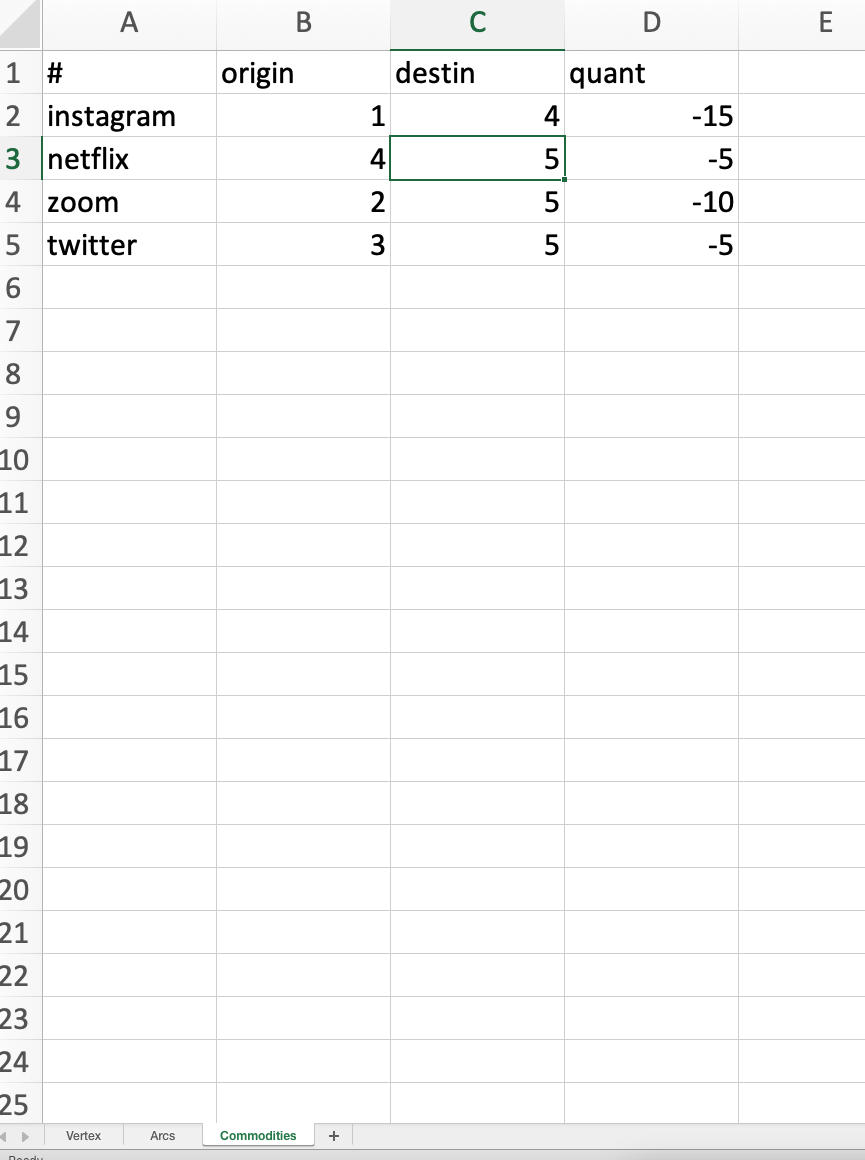# Add a vertex to the set of vertices and the graphimport networkx as netx  # nice (di-)graph Python packageimport matplotlib.pyplot as pltimport gurobipy as gpimport xlrdvertices = []vertices_no = 0weight = []graph = []leavingnode=[]comingnode=[]price ={}maxcapacity=[]comm=[]tran=[]demand ={}start=[]cnode=[]nodes=[]G = netx.DiGraph()var_names = []var_values = []demands={}commo=[]origin=[]destination=[]dem=[]loc1 = ("/Users/fer/PycharmProjects/project/Input_lecct copy.xls")wb = xlrd.open_workbook(loc1)Vertex=wb.sheet_by_index(0)Arcs=wb.sheet_by_index(1)Commodities=wb.sheet_by_index(2)################ adding vertex from excel ######################def add_vertex(v):    global graph    global vertices_no    global vertices    if v in vertices:        print("Vertex ", v, " already exists")    else:        vertices_no = vertices_no + 1        vertices.append(v)        if vertices_no > 1:            for vertex in graph:                vertex.append(0)        temp = []        for i in range(vertices_no):            temp.append(0)        graph.append(temp)################ adding vertex from excel ######################def add_edge(v1, v2,e):    global graph    global vertices_no    global vertices    # Check if vertex v1 is a valid vertex    if v1 not in vertices:        print("Vertex ", v1, " does not exist.")    # Check if vertex v1 is a valid vertex    elif v2 not in vertices:        print("Vertex ", v2, " does not exist.")    # Since this code is not restricted to a directed or    # an undirected graph, an edge between v1 v2 does not    # imply that an edge exists between v2 and v1    else:        index1 = vertices.index(v1)        index2 = vertices.index(v2)        graph[index1][index2] = e################ print the pair of nodes and edgeweight ######################def print_graph():    global graph    global vertices_no    for i in range(vertices_no):        for j in range(vertices_no):            if graph[i][j] != 0:                print(vertices[i], " -> ", vertices[j], \                      " edge weight: ", graph[i][j])                #print verticesdef draw_graph():    global graph    global vertices_no    global vertices    global weight    for i in range(vertices_no):        for j in range(vertices_no):            if graph[i][j] != 0:                weight=(graph[i][j])                G.add_edge(vertices[i], vertices[j], weight=weight)    epos = [(u, v) for (u, v, d) in G.edges(data=True) if d["weight"] > 0]    pos = netx.spring_layout(G, k=150, seed=200)  # positions for all nodes - seed for reproducibility    # nodes    netx.draw_networkx_nodes(G, pos)    # edges    labels = netx.get_edge_attributes(G, 'weight')    netx.draw_networkx_edge_labels(G, pos, edge_labels=labels)    netx.draw_networkx_edges(G, pos, edgelist=epos, width=10,edge_color="orange")    # labels    netx.draw_networkx_labels(G, pos, font_size=20, font_family="sans-serif")    ax = plt.gca()    ax.margins(0.08)    plt.axis("off")    #plt.tight_layout()    plt.show()    #plt.savefig("graphp.png")################ multicommodity######################def max_flow(commodity,leavingnode,commingnode, origin,destination,cost,demandz):    global graph    global vertices_no    global vertices    global weight    listkeyPair=[]    for i in range(vertices_no):        for j in range(vertices_no):           if graph[i][j] != 0:                listkeyPair.append((vertices[i], vertices[j]))    print("This prints out the nodes in the pair: ",listkeyPair)    listvalueCap=[]    for i in range(vertices_no):        for j in range(vertices_no):            if  graph[i][j] != 0:                listvalueCap.append((graph[i][j]))    print("This prints out the capacity of the node pair: ", listvalueCap)    pair,capacity = gp.multidict(dict(zip(listkeyPair, listvalueCap)))    print("The dictionary after the merge of the pairs of nodes with the capacities:")    print (capacity)    listnodes=[]    for i in range(vertices_no):                listnodes.append((commodity,vertices[i]))    print("lsit of nodes with each commodity:", listnodes)    listactualnodes = []    for i in range(vertices_no):            listactualnodes.append((vertices[i]))    print("lsit of actual nodes:", listactualnodes)    nodes =leavingnode +commingnode    print("list of all incoming and outgoingnodes: ", nodes)    # Create optimization model    m = gp.Model('netflow')    # Create variables    # flow = m.addVars(test,di, obj=cost, name="flow")    flow = m.addVars(commodity, pair, obj=cost,vtype = "C", name="flow")    # m.setObjective(flow.prod(cost), GRB.MINIMIZE)    m.update()    # Arc-capacity constraints    for x, y in pair:        m.addConstr(sum(flow[h, x, y] for h in commodity) <= capacity[x, y], "cap[%s, %s]" % (x, y))    """    m.addConstrs(        (gp.quicksum(flow[h, x, y] for x, y in pair.select('*', y)) + demandz[h,o,d] ==         gp.quicksum(flow[h, y, k] for y, k in pair.select(y, '*'))         for h in commodity for o in origin for d in destination), "node")    """    for h in commodity:        for y in nodes:          for a in range(len(commodity)):            if y == origin[a]:                print("test 1")                m.addConstrs(                    ((gp.quicksum(flow[h, y, x] for x in leavingnode ) - gp.quicksum(                        flow[h, x, y] for x in comingnode )) == abs(demandz[h,o,d])                     for h in commodity for o in origin for d in destination ), name = 'Continuity(%s, %s)' % (h, y))            elif y == destination[a]:                print("test 2")                m.addConstrs(                    ((gp.quicksum(flow[h, y, x] for  x in leavingnode ) - gp.quicksum(                        flow[h, x, y] for x in commingnode )) == demandz[h,o,d]                     for h in commodity for o in origin for d in destination) , name= 'Continuity(%s, %s)' % (h, y))            else:                print("test 3")                m.addConstrs(                    ((gp.quicksum(flow[h, y, x] for  x in leavingnode ) - gp.quicksum(                        flow[h, x, y] for  x in commingnode ) ) == 0                 ), name= 'Continuity(%s, %s)' % (h, y))    # Compute optimal solutions    m.optimize()    print (m.display())    print("if model fesible it will print a 2 .", m.status)    # Print solution    # print solution: optimal value and optimal point    print('Obj: %g' % m.objVal)     #print(f"optimal value = {model.objVal:.2f}")    for var in m.getVars():       #print('%s %g' % (v.varName, v.x))       if var.X > 0:           var_names.append(str(var.varName))           var_values.append(var.X)#################Driver##########################adding the vertex sheet with all the nodesp = 1while True:    try:        v = Vertex.cell_value(p, 0)        #adds it to list of vertices        add_vertex(v)        #adds it to draw graph        nodes.append(v)        print(nodes)        p = p+1    except IndexError:        break# adding the arcs sheet with i, j,capacity  and then matching them with a costi = 1while True:    try:        l = Arcs.cell_value(i, 0)        leavingnode.append(l)        c = Arcs.cell_value(i, 1)        comingnode.append(c)        cap = Arcs.cell_value(i,3)        maxcapacity.append(cap)        add_edge(l,c,cap)        i = i+1    except IndexError:        breakz = 1while True:    try:        for leave  in leavingnode:           c = Arcs.cell_value(z, 1)           price[leave,c]=Arcs.cell_value(z,2)           print("cost:", price[leave,c])           z = z+1    except IndexError:        break# adding the commodities sheet with #, origin, destination and quantityi = 1while True:    try:        item= Commodities.cell_value(i,0)        commo.append(item)        ori = Commodities.cell_value(i, 1)        origin.append(ori)        dest = Commodities.cell_value(i, 2)        destination.append(dest)        i = i+1    except IndexError:        breaka = 1while True:    try:        for it in commo:           ori = Commodities.cell_value(a, 1)           dest = Commodities.cell_value(a, 2)           dem.append(demands)           demands[it,ori,dest]=Commodities.cell_value(a,3)           print("Demands:", demands[it,ori,dest])           a = a+1    except IndexError:        breakprint_graph()print("Internal representation: ", graph)#draw_graph()print("END:")print("commo",commo)print("origin:",origin)print('destination',destination)print("leaving", leavingnode)print('coming', comingnode)max_flow(commo,leavingnode,comingnode, origin,destination, price, demands)
•Gurobi Staff

Hi Fernanda,

The error is that you access $$\texttt{flow[instagram, 1.0, 1.0]}$$ which is not present.

for y in nodes:  for a in range(len(commodity)):    if y == origin[a]:      print("test 1")        m.addConstrs(        ((gp.quicksum(flow[h, y, x] for x in leavingnode ) - gp.quicksum(         flow[h, x, y] for x in commingnode )) == abs(demandz[h,o,d])         for h in commodity for o in origin for d in destination ), name = 'Continuity(%s, %s)' % (h, y))

You iterate $$\texttt{y}$$ over all $$\texttt{nodes}$$, so in the first iteration $$\texttt{y=1.0}$$. The list $$\texttt{leavingnode}$$ is

[1.0, 1.0, 2.0, 2.0, 3.0, 3.0, 4.0]

Thus, you try to access $$\texttt{flow[instagram, 1.0, 1.0]}$$, which is not present. You should rather iterate over all arcs ($$\texttt{pair}$$ in your code).

You can print the lists $$\texttt{nodes, commingnode, leavingnode}$$ to check which variable is iterated over which values.

Moreover, there is a typo $$\texttt{comingnode}$$ should read $$\texttt{commingnode}$$ in line 193.

Best regards,
Jaromił

•Hello Jaromil,

thanks again so I changed it slightly but now the issue is the syntax for m.Constrs or m.Constr. When I do m.Constrs , I get 3 printed results. When I do m.Constr, we get one printed result and the error says that its missing a constraint index. Not sure which one to use because they both seem to be giving an error.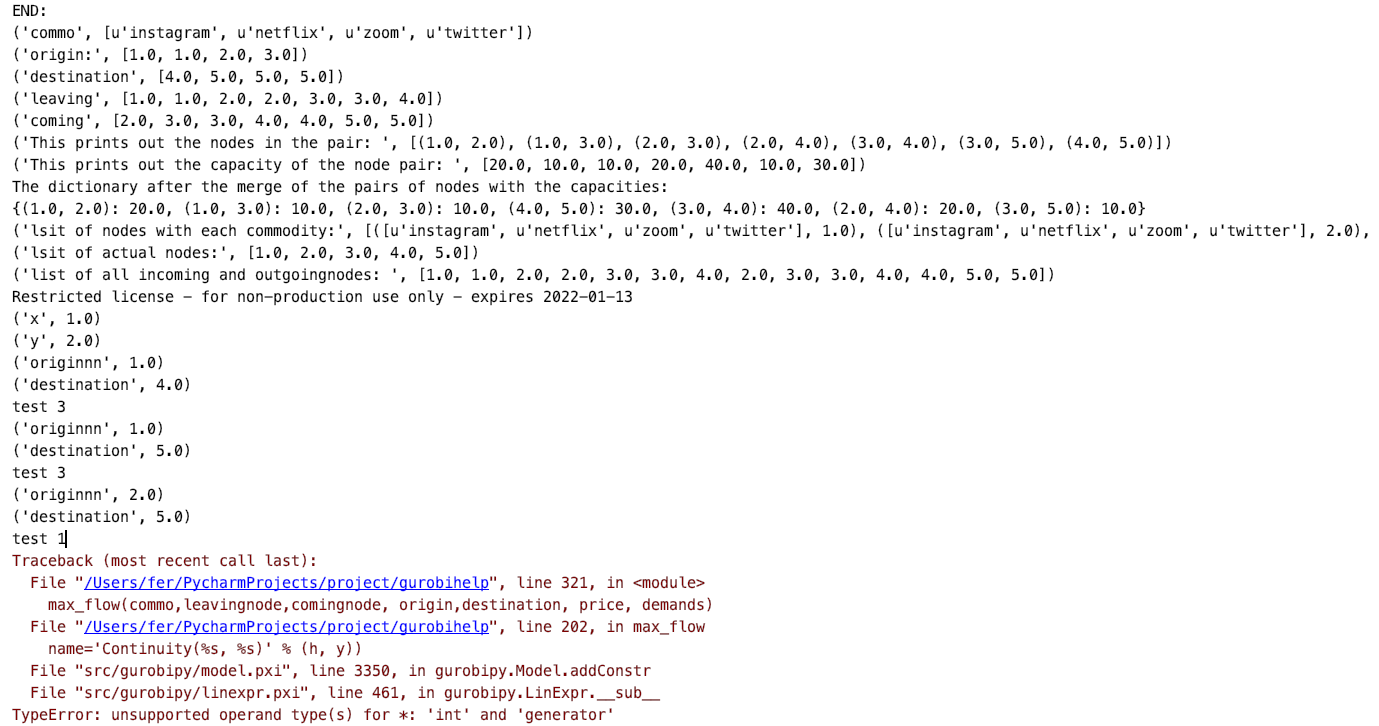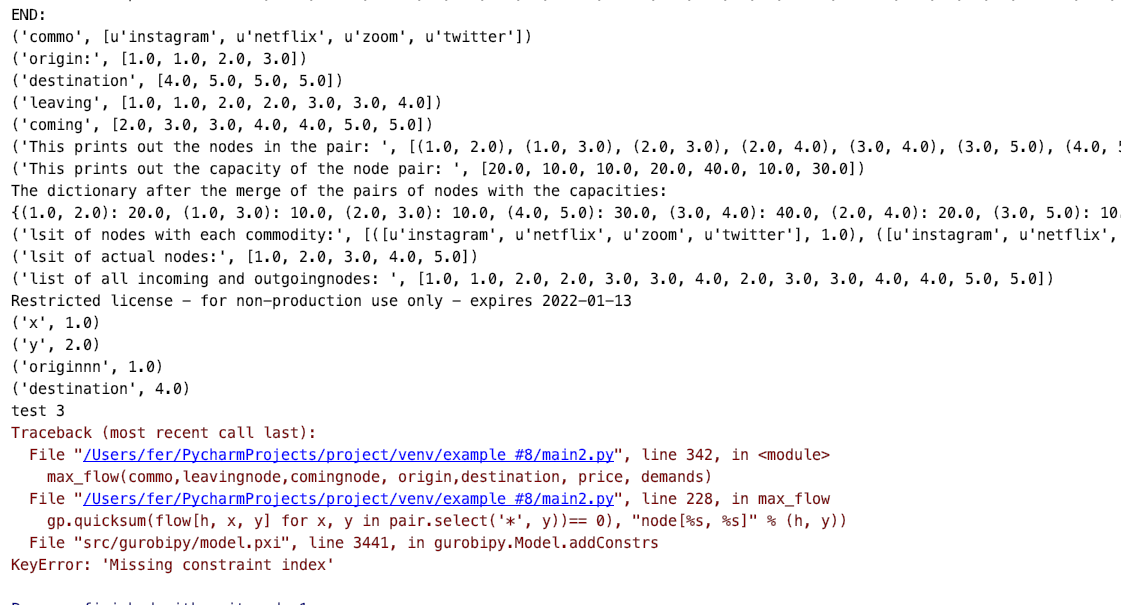•Gurobi Staff

Hi Fernanda,

addConstrs needs a generator expression to construct multiple constraints at once.

Thus, the code

 m.addConstrs(((gp.quicksum(flow[h, y, x] for  x in leavingnode ) - gp.quicksum(               flow[h, x, y] for  x in commingnode ) ) == 0),               name= 'Continuity(%s, %s)' % (h, y))

is incorrect, because you don't generate multiple constraints but rather just one. So in this case, you should use addConstr. The above should read

 m.addConstr(((gp.quicksum(flow[h, y, x] for x in leavingnode ) - gp.quicksum(               flow[h, x, y] for x in commingnode ) ) == 0),               name= 'Continuity(%s, %s)' % (h, y))

Please note that the code above is using your old code. Could you provide the changes you made to avoid the key error? Are you now able to run your code successfully?

Best regards,
Jaromił

•Hello again,

yes so if we also change it to addConstr, it still gives us an error (screenshot below).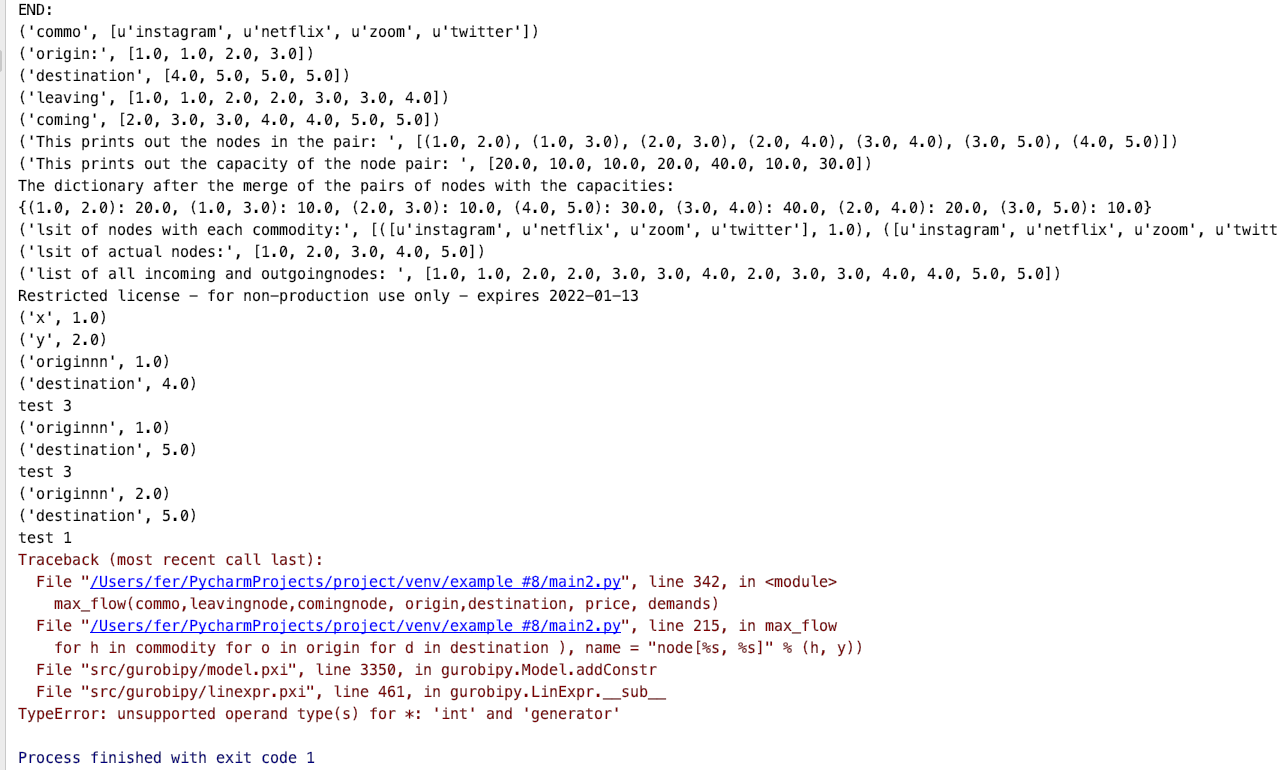We provided the most recent code below as well.

import networkx as netx import matplotlib.pyplot as pltimport gurobipy as gpimport xlrdvertices = []vertices_no = 0weight = []graph = []leavingnode=[]comingnode=[]price ={}maxcapacity=[]comm=[]tran=[]demand ={}start=[]cnode=[]nodes=[]G = netx.DiGraph()var_names = []var_values = []demands={}commo=[]origin=[]destination=[]dem=[]loc1 = ("/Users/fer/PycharmProjects/project/Input_lecct copy.xls")wb = xlrd.open_workbook(loc1)Vertex=wb.sheet_by_index(0)Arcs=wb.sheet_by_index(1)Commodities=wb.sheet_by_index(2)################ adding vertex from excel ######################def add_vertex(v):    global graph    global vertices_no    global vertices    if v in vertices:        print("Vertex ", v, " already exists")    else:        vertices_no = vertices_no + 1        vertices.append(v)        if vertices_no > 1:            for vertex in graph:                vertex.append(0)        temp = []        for i in range(vertices_no):            temp.append(0)        graph.append(temp)################ adding vertex from excel ######################def add_edge(v1, v2,e):    global graph    global vertices_no    global vertices    # Check if vertex v1 is a valid vertex    if v1 not in vertices:        print("Vertex ", v1, " does not exist.")    # Check if vertex v1 is a valid vertex    elif v2 not in vertices:        print("Vertex ", v2, " does not exist.")    # Since this code is not restricted to a directed or    # an undirected graph, an edge between v1 v2 does not    # imply that an edge exists between v2 and v1    else:        index1 = vertices.index(v1)        index2 = vertices.index(v2)        graph[index1][index2] = e################ print the pair of nodes and edgeweight ######################def print_graph():    global graph    global vertices_no    for i in range(vertices_no):        for j in range(vertices_no):            if graph[i][j] != 0:                print(vertices[i], " -> ", vertices[j], \                      " edge weight: ", graph[i][j])                #print verticesdef draw_graph():    global graph    global vertices_no    global vertices    global weight    for i in range(vertices_no):        for j in range(vertices_no):            if graph[i][j] != 0:                weight=(graph[i][j])                G.add_edge(vertices[i], vertices[j], weight=weight)    epos = [(u, v) for (u, v, d) in G.edges(data=True) if d["weight"] > 0]    pos = netx.spring_layout(G, k=150, seed=200)  # positions for all nodes - seed for reproducibility    # nodes    netx.draw_networkx_nodes(G, pos)    # edges    labels = netx.get_edge_attributes(G, 'weight')    netx.draw_networkx_edge_labels(G, pos, edge_labels=labels)    netx.draw_networkx_edges(G, pos, edgelist=epos, width=10,edge_color="orange")    # labels    netx.draw_networkx_labels(G, pos, font_size=20, font_family="sans-serif")    ax = plt.gca()    ax.margins(0.08)    plt.axis("off")    #plt.tight_layout()    plt.show()    #plt.savefig("graphp.png")################ multicommodity######################def max_flow(commodity,leavingnode,commingnode, origin,destination,cost,demandz):    global graph    global vertices_no    global vertices    global weight    listkeyPair=[]    for i in range(vertices_no):        for j in range(vertices_no):           if graph[i][j] != 0:                listkeyPair.append((vertices[i], vertices[j]))    print("This prints out the nodes in the pair: ",listkeyPair)    listvalueCap=[]    for i in range(vertices_no):        for j in range(vertices_no):            if  graph[i][j] != 0:                listvalueCap.append((graph[i][j]))    print("This prints out the capacity of the node pair: ", listvalueCap)    pair,capacity = gp.multidict(dict(zip(listkeyPair, listvalueCap)))    print("The dictionary after the merge of the pairs of nodes with the capacities:")    print (capacity)    listnodes=[]    for i in range(vertices_no):                listnodes.append((commodity,vertices[i]))    print("lsit of nodes with each commodity:", listnodes)    listactualnodes = []    for i in range(vertices_no):            listactualnodes.append((vertices[i]))    print("lsit of actual nodes:", listactualnodes)    nodes =leavingnode +commingnode    print("list of all incoming and outgoingnodes: ", nodes)    # Create optimization model    m = gp.Model('netflow')    # Create variables    # flow = m.addVars(test,di, obj=cost, name="flow")    flow = m.addVars(commodity, pair, obj=cost,vtype = "C", name="flow")    # m.setObjective(flow.prod(cost), GRB.MINIMIZE)    m.update()    # Arc-capacity constraints    for x, y in pair:        m.addConstr(sum(flow[h, x, y] for h in commodity) <= capacity[x, y], "cap[%s, %s]" % (x, y))     for h in commodity:        for x, y in pair:            print("x", x)            print("y", y)            for a in range(len(commodity)):                print ("originnn", origin[a])                print ("destination", destination[a])                if y == origin[a]:                    print("test 1")                    m.addConstr(                        gp.quicksum(flow[h, y, k] for y, k in pair.select(y, '*')) -                        gp.quicksum(flow[h, x, y] for x, y in pair.select('*', y)), '=', (abs(demandz[h, o, d])                                                                                          for h in commodity for o in                                                                                          origin for d in destination),                        name="node[%s, %s]" % (h, y))                elif y == destination[a]:                    print("test 2")                    m.addConstr(                        gp.quicksum(flow[h, y, k] for y, k in pair.select(y, '*')) -                        gp.quicksum(flow[h, x, y] for x, y in pair.select('*', y)), '=', (demandz[h, o, d]                                                                                          for h in commodity for o in                                                                                          origin for d in destination),                        name="node[%s, %s]" % (h, y))                else:                    print("test 3")                    m.addConstr(                        gp.quicksum(flow[h, y, k] for y, k in pair.select(y, '*')) -                        gp.quicksum(flow[h, x, y] for x, y in pair.select('*', y)), '=', 0,                        name="node[%s, %s]" % (h, y))    # Compute optimal solutions    m.optimize()    print (m.display())    print("if model fesible it will print a 2 .", m.status)    # Print solution    # print solution: optimal value and optimal point    print('Obj: %g' % m.objVal)     #print(f"optimal value = {model.objVal:.2f}")    for var in m.getVars():       #print('%s %g' % (v.varName, v.x))       if var.X > 0:           var_names.append(str(var.varName))           var_values.append(var.X)#################Driver##########################adding the vertex sheet with all the nodesp = 1while True:    try:        v = Vertex.cell_value(p, 0)        #adds it to list of vertices        add_vertex(v)        #adds it to draw graph        nodes.append(v)        print(nodes)        p = p+1    except IndexError:        break# adding the arcs sheet with i, j,capacity  and then matching them with a costi = 1while True:    try:        l = Arcs.cell_value(i, 0)        leavingnode.append(l)        c = Arcs.cell_value(i, 1)        comingnode.append(c)        cap = Arcs.cell_value(i,3)        maxcapacity.append(cap)        add_edge(l,c,cap)        i = i+1    except IndexError:        breakz = 1while True:    try:        for leave  in leavingnode:           c = Arcs.cell_value(z, 1)           price[leave,c]=Arcs.cell_value(z,2)           print("cost:", price[leave,c])           z = z+1    except IndexError:        break# adding the commodities sheet with #, origin, destination and quantityi = 1while True:    try:        item= Commodities.cell_value(i,0)        commo.append(item)        ori = Commodities.cell_value(i, 1)        origin.append(ori)        dest = Commodities.cell_value(i, 2)        destination.append(dest)        i = i+1    except IndexError:        breaka = 1while True:    try:        for it in commo:           ori = Commodities.cell_value(a, 1)           dest = Commodities.cell_value(a, 2)           dem.append(demands)           demands[it,ori,dest]=Commodities.cell_value(a,3)           print("Demands:", demands[it,ori,dest])           a = a+1    except IndexError:        breakprint_graph()print("Internal representation: ", graph)#draw_graph()print("END:")print("commo",commo)print("origin:",origin)print('destination',destination)print("leaving", leavingnode)print('coming', comingnode)max_flow(commo,leavingnode,comingnode, origin,destination, price, demands)
•Hello again,

I still haven't heard back, but it finally prints out results but now the problem is that it repeats the same set of vertices for each commodity about 7 times. Here are some screenshots. please let me know what the issue is.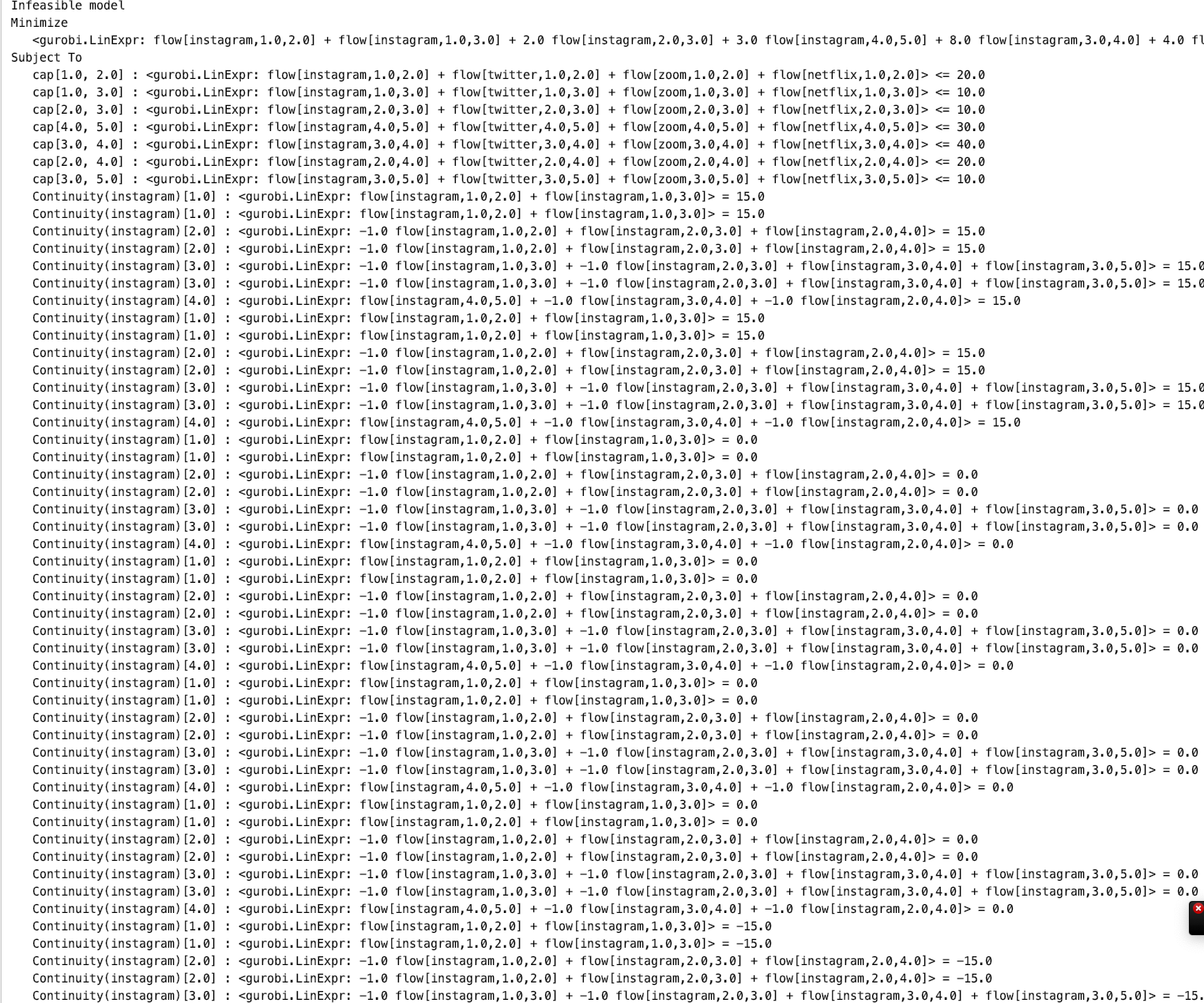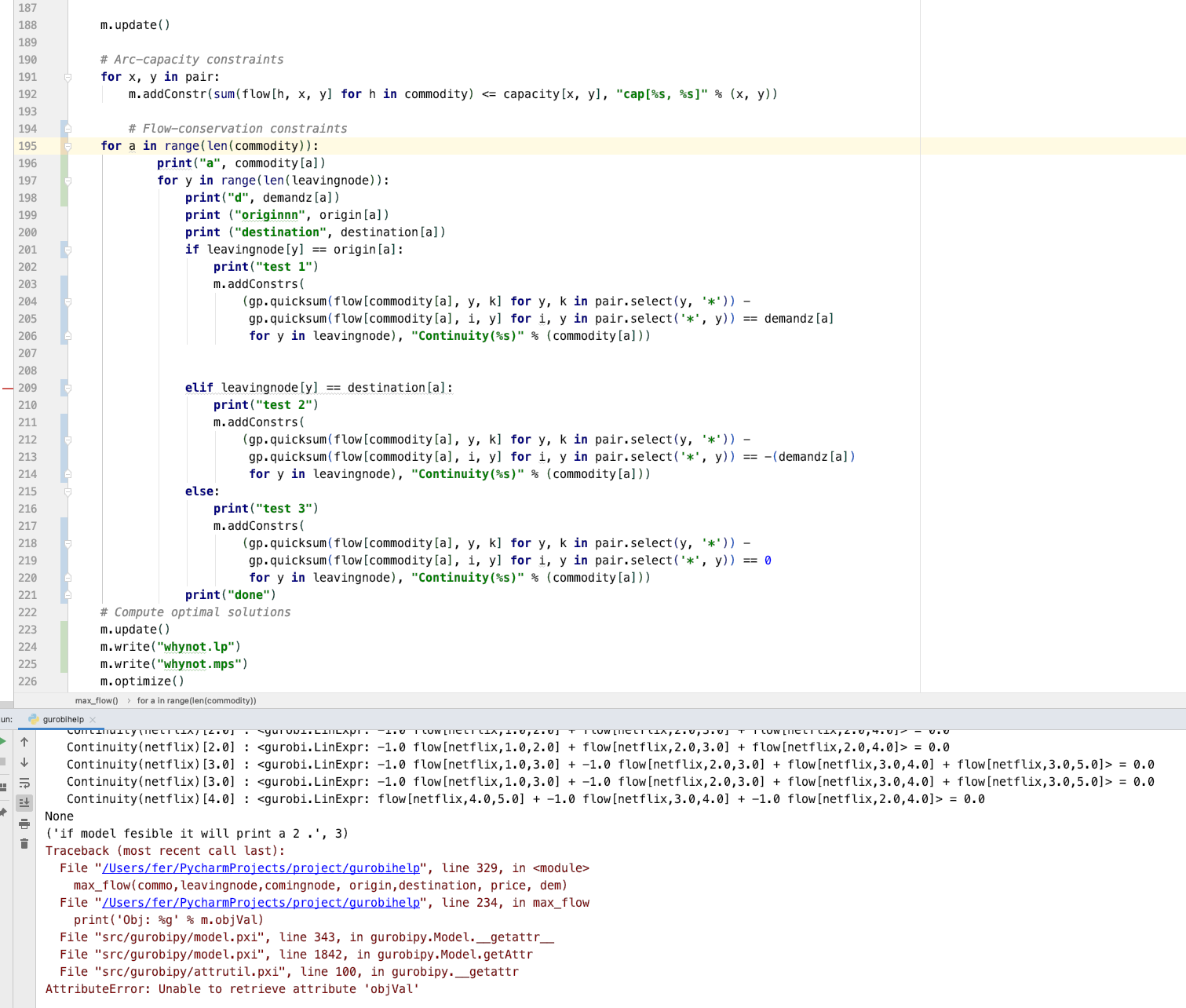•Gurobi Staff

Hi Fernanda,

Sorry for getting back to you, I was out for the last weeks.

The issue may be caused by using $$\texttt{y}$$ as an iterator multiple times in your $$\texttt{for}$$-loops.

$$\texttt{y}$$ is used in

for y in range(len(leavingnode)):

and then again in multiple contexts in the

m.addConstrs(... for y, k ... for y in leavingnode ...)

terms.

Please also note that the model status you get in your last screenshot is 3 which means $$\texttt{INFEASIBLE}$$. Thus, you cannot access the ObjVal attribute. You could use the write() function to write an LP file and analyze by hand whether the model looks as expected

m.write("myLP.lp")

Best regards,
Jaromił